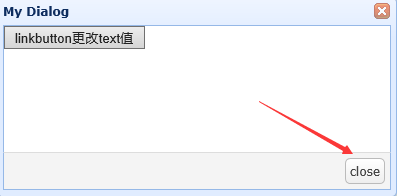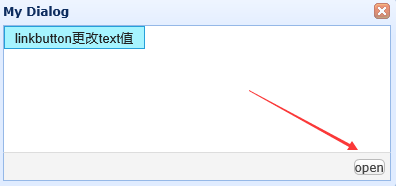`````` \$('#dd').dialog({
title: 'My Dialog',
width: 400,
height: 200,
closed: false,
cache: false,
href: 'test.html',
modal: true,
buttons : [
{
text : 'close',
id :'expandCollapse'
}
]
});
\$('#dd').dialog();
```````````` function expandCollapseAll(){
var type = \$('#expandCollapseAll').value;
if (type == 1){
\$('#resourceTree').tree('expandAll');//展开所有
\$('#expandCollapseAll').value = 0;
\$('#expandCollapse').text = 'close';
}else if (type == 0){
\$('#resourceTree').tree('collapseAll');//折叠所有
\$('#expandCollapseAll').value = 1;
\$('#expandCollapse').text = 'open';
}
}
``````

2个回答

`````` function expandCollapseAll(){
var type = \$('#expandCollapseAll').value;
if (type == 1){
\$('#resourceTree').tree('expandAll');//展开所有
\$('#expandCollapseAll').value = 0;
//\$('#expandCollapse').text = 'close';
}else if (type == 0){
\$('#resourceTree').tree('collapseAll');//折叠所有
\$('#expandCollapseAll').value = 1;
//\$('#expandCollapse').text = 'open';